# Gradient surfing — the hidden role of regularization

Produced as part of the SERI ML Alignment Theory Scholars Program - Winter 2022 Cohort

In a previous post, I demonstrated that Brownian motion near singularities defies our expectations from "regular" physics. Singularities trap random motion and take up more of the equilibrium distribution than you'd expect from the Gibbs measure.

In the computational probability community, this is a well-known pathology. Sampling techniques like Hamiltonian Monte Carlo get stuck in corners, and this is something to avoid. You typically don't want biased estimates of the distribution you're trying to sample.

In deep learning, I argued, this behavior might be less a bug than a feature.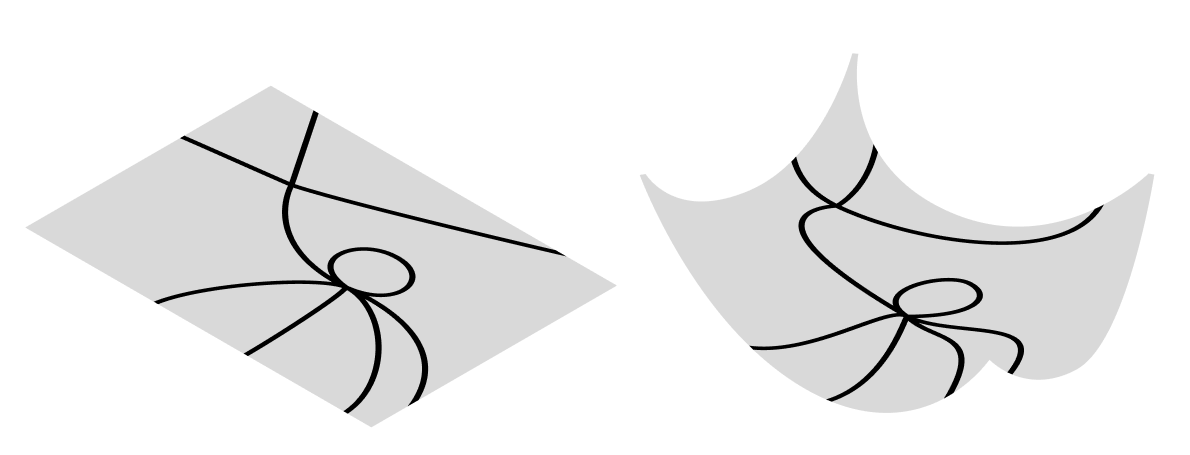Regularization may have a hidden function to make the set of minimum-loss points more navigable. Simply drifting around this set of points privileges simple solutions, even in the absence of explicit complexity penalties. Or not. The evidence isn't conclusive.

The claim of singular learning theory is that models near singularities have lower effective dimensionality. From Occam's razor, we know that simpler models generalize better, so if the dynamics of SGD get stuck at singularities, it would suggest an explanation (at least in part) for why SGD works: the geometry of the loss landscape biases your optimizer towards good solutions.

This is not a particularly novel claim. Similar versions of the claim been made before by Mingard et al. and Valle Pérez et al.. But from what I can tell, the proposed mechanism, of singularity "stickiness", is quite different.

Moreover, it offers a new possible explanation for the role of regularization. If exploring the set of points with minimum training loss is enough to get to generalization, then perhaps the role of regularizer is not just to privilege "simpler" functions but also to make exploration possible.

In the absence of regularization, SGD can't easily move between points of equal loss. When it reaches the bottom of a valley, it's pretty much stuck. Adding a term like weight decay breaks this invariance. It frees the neural network to surf the loss basin, so it can accidentally stumble across better generalizing solutions.

So could we improve generalization by exploring the bottom of the loss basin in other ways — without regularization or even without SGD? Could we, for example, get a model to grok through random drift?

…No. We can't.

That is to say I haven't succeeded yet. Still, in the spirit of "null results are results", let me share the toy model that motivated this hypothesis and the experiments that have (as of yet) failed to confirm it.

# The inspiration: a toy model

First, let's take a look at the model that inspired the hypothesis.

Let's begin by modifying the example of the previous post to include an optional regularization term controlled by $\lambda$:

$U(\boldsymbol x) = a\left(\min(x_0^2,\ x_1^2)\right) + \frac{\lambda}{2} (\boldsymbol x - \boldsymbol c) ^2.$

We deliberately center the regularization away from the origin at $\boldsymbol c = (-1,\, -1)$ so it doesn't already privilege the singularity at the origin.

Now, instead of viewing $U(\boldsymbol x)$ as a potential and exploring it with Brownian motion, we'll treat it as a loss function and use stochastic gradient descent to optimize for $\boldsymbol x$.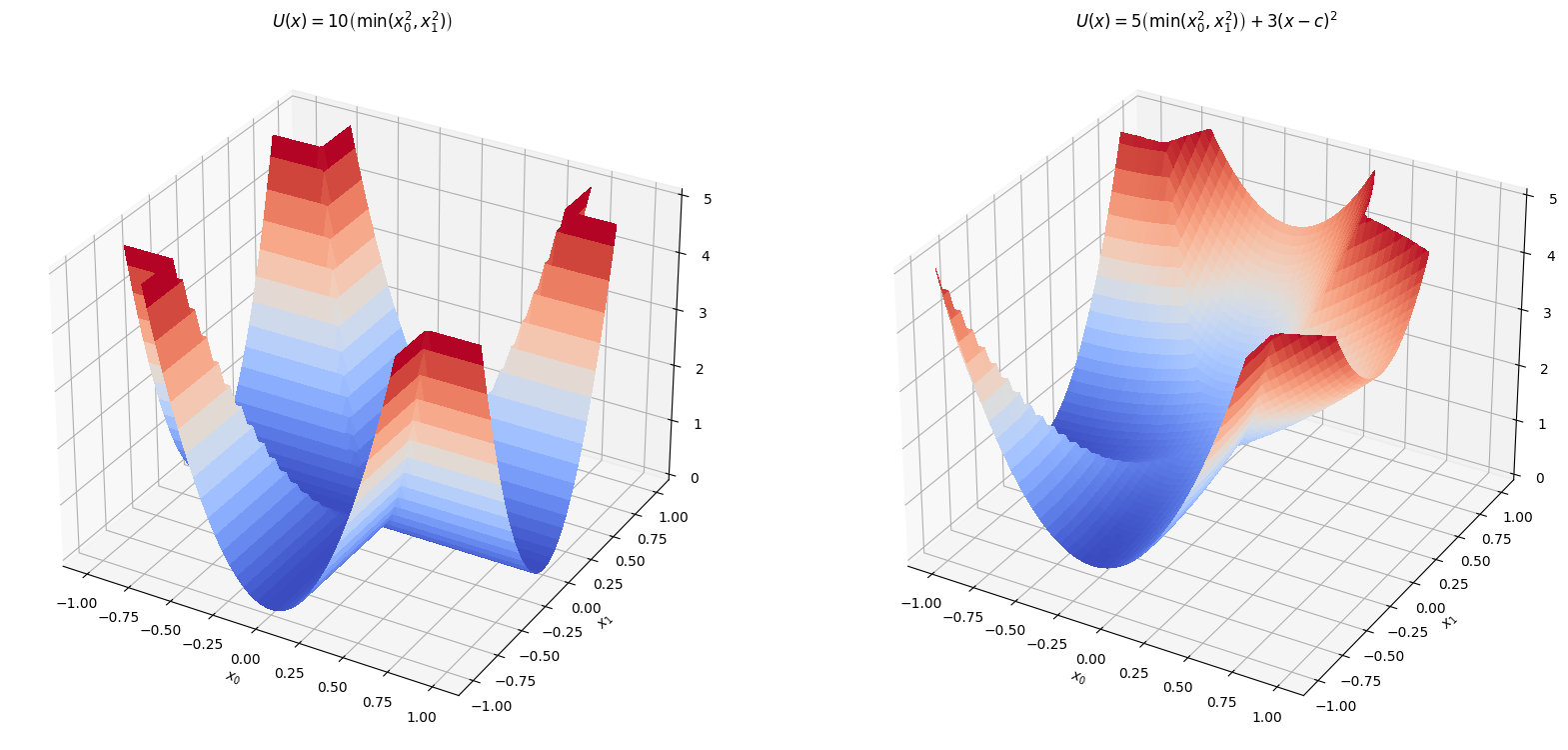Including regularization breaks the symmetry of the minimum loss set. Unlike before, we'll remove the toroidal boundary conditions (to avoid nasty discontinuities at the boundaries).

We'll start our optimizer at a uniformly sampled random point in this region and take $T=100$ steps down the gradient (with optional momentum controlled by $\beta$). After each gradient step, we'll inject a bit of Gaussian noise to simulate the "stochasticity." Altogether, the update rule for $\boldsymbol x$ is as follows:

$\boldsymbol x^{(t+1)} \leftarrow \boldsymbol x^{(t)} + \eta \ \boldsymbol v^{(t+1)}+ \boldsymbol \delta^{(t)},$

with momentum updated according to:

$\boldsymbol v^{(t+1)} \leftarrow \beta \cdot\boldsymbol v^{(t)} + (1-\beta)\cdot (-\nabla_{\boldsymbol x} U),$

and noise given by,

$\delta_i^{(t)} \sim \mathcal N(0, \epsilon^2).$

If we sample the final obtained position, $\boldsymbol x^{(T)}$ over independent initializations, then, in the absence of regularization and in the presence of a small noise term, we'll get a distribution that looks like the figure on the left.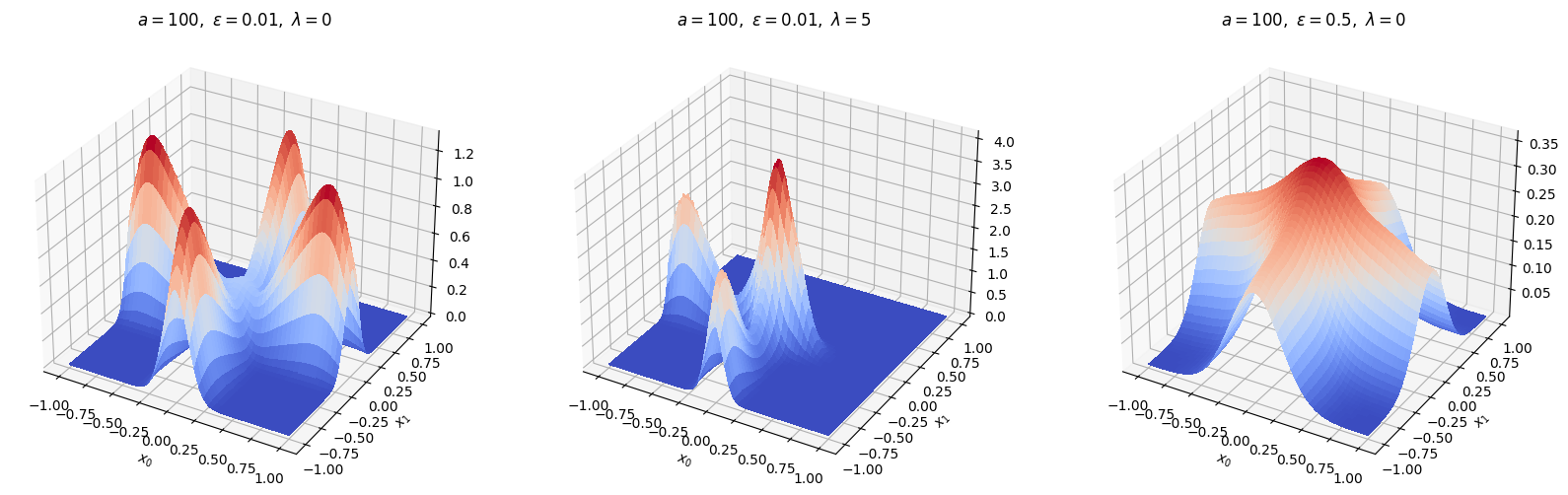Under vanilla SGD, the singularity becomes repulsive (at least for this toy model). We can recover the stickiness of the singularity by increasing the amount of noise or adding a (relatively) small regularization term.

Unlike the case of random motion, the singularity at the origin is now repulsive. Good luck finding those simple solutions now.

However, as soon as we turn on the regularization (middle figure) or increase the noise term (figure on the right), the singularity once again becomes favored. This is true even though the origin no longer minimizes the overall loss.

So noise and regularization1 bias SGD towards singularities. If you buy that singularities correspond to simpler solutions, this might mean a novel, unexplored2 inductive bias towards generalization.

# Grokking through random motion

Let's test this hypothesis in a more realistic setting.

Here's one application: can we use random drift at the bottom of the loss basin to induce grokking?3 In other words, given a model with low training error but high test error, can we improve test performance just by increasing the amount of "lateral" motion (that preserves rather than lowers loss).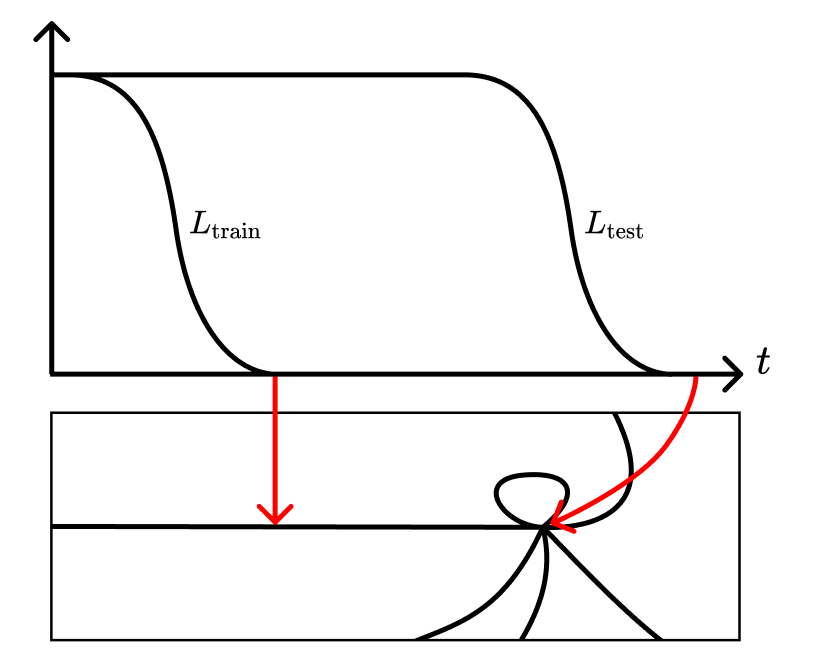Could we grok simply by exploring the set of minimum loss points and getting "stuck" at good solutions?

In particular, can we use noise to make a model grok even in the absence of regularization (which is currently a requirement to make models grok with SGD)?

If this were true, it wouldn't give us enough evidence to distinguish the contribution of singularities from other factors like simple functions taking up more weight-space volume, but it'd be a start.

Unfortunately, it seems pretty difficult to grok without regularization.4

Some of the techniques I've used to explore the loss basin have had minor success (up to a $\sim 5\%$ sustained bump in test accuracy over Adam without weight decay). But nothing approaches the sustained grokking of Adam with weight decay.

## Weird regularizations and additional noise

One way to encourage lateral motion is to abuse the regularization term. I tried both a repulsive variant (where I reward the model for displacement from its SGD-primed starting point) and a variant that selected for large weight norms (by punishing distance from a larger weight norm). I also tried a variant that simply adds extra noise after each gradient step.

All of these improve test performance somewhat. What seems to happen is that these variants dislodge the model from a very fragile initial equilibrium to find a new slightly better and more stable solution. It outperforms Adam without weight decay but quickly plateaus (and, in the case of "antiregularization", slowly declines after reaching a peak).

Of the three, antiregularization is able to reach the highest improvements in accuracy (up to 40%), but the improvements are unstable, and final performance is worse than it started out. Still, the fact that improving generalization is at all possible while increasing weight norm is intriguing. And the fact that the variance in performance is so high could be evidence that favors an explanation in terms of singularities over flat basins, but high-dimensional spaces are weird, and the results are anything but conclusive.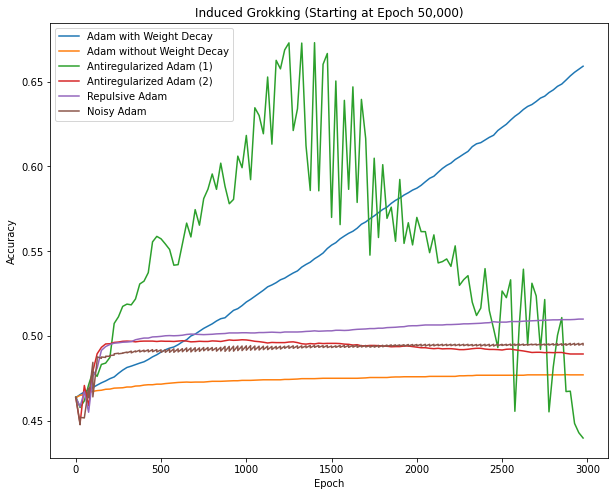Note: these variants only work for small-to-zero momentum, and they work best near the inflection point of $50\%$ test accuracy.

## Hamiltonian Monte Carlo

Another idea to explore the set of minimum loss points is to steal from physics: the trick behind Hamiltonian Monte Carlo is to view the loss as a potential energy and then to simulate a physical particle moving through that potential energy landscape.Hamiltonian Monte Carlo turns your sampling problem into a physics simulation [source].

From the equipartition theorem, we can associate the starting loss to an inverse temperature, which describes a physical system with that loss as its average.

The system will still accept changes that decrease the loss, but it will also occasionally accept changes that increase the loss. Over the long run, these two forces will balance out, so the expected loss remains constant.

$\overset{\Large \text{Hyperparameters of Hamiltonian Monte Carlo}}{ \begin{array}{c c c c} \\ \hline \text{Symbol }& \text{Hyperparameter } & \text{Role} \\ \hline \beta& \text{Inverse Temperature} & \text{Controls probability of accepting an increase in loss.}\\ \lambda & \text{Weight Decay} & \text{Controls balance between loss \& regularization}. \\ \eta\ (\text{or }\delta t)& \text{Learning Rate} & \text{Controls size of each step.} \\ m & \text{Mass} & \text{Controls relative step-size of momentum and weights.} \\ L & \text{Check Interval} & \text{Number of steps between Metropolis-Hastings checks.} \end{array}}$

Though this would be the "cleanest" form of exploration (it is precisely the Brownian motion discussed previously), it doesn't seem to work (regardless of the choice of hyperparameters and of where I start HMC along the grokking curve).

Then again, I also can't get this to work even in the presence of regularization. In this case, by lowering the temperature, HMC should limit to a slow version of gradient descent. The code seems right, so I'm inclined to write it off to high-dimensional spaces being hard and my compute budget being limited.

## What does this mean for singularities?

The negative result tells us that the strong form of the claim "regularization = navigability" is probably wrong. Having a smaller weight norm actually is good for generalization (just as the learning theorists would have you believe). You'll have better luck moving along the set of minimum loss weights in the way that minimizes the norm than in any other way.

But the observation that you can, for a time, increase generalization performance by selecting for much larger norms suggests we can't outright reject the weaker version of the claim. Simply exploring the minimum loss set may — still — privilege simpler solutions.

## Footnotes

1. Momentum doesn't have much of an effect on this example.

2. From what I've seen so far.